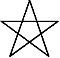There are eight months in the Calendar of Mu, sometimes referred to as the Nyarzirian Calendar. Each month is consists of forty-five days, and represents a system of the eight hexagrams moving through the five elements. The Ninzuwu Priest/Priestess invokes the mudra/mantra formulae associated with each hexagram that rules a particular day after invoking the Armor of Amaterasu Ohkami. The chart below illustrates the daily Vasuh letters invoked and the ruling “deity” for the said hexagram:
1. March 25th, 2015 = Hahun = 14th Hexagram = Zhee-Bnhu-Aum
2. March 26th, 2015 = Shapash = 21st Hexagram =Phe-Aum-Nzu
3. March 27th 2015 = Amaterasu = 30th Hexagram = Zhee-Lewhu
4. March 28th 2015 = Nuru = 35th Hexagram = Bnhu
5. March 29th 2015 = Yu Nin = 38th Hexagram = Phe-Aum-Shki
6. March 30th 2015 = Kagutsuchi = 50th Hexagram = Hmu-Hmu
7. March 31st 2015 = Fujiyama = 56th Hexagram = Bnhu-Phe
8. April 1st 2015 = Tengu = 64th Hexagram = Zhee-Tuu-Zhee-Hmu
9. April 2nd 2015 =Entering Earth Element
10. April 3rd 2015 = Hahun = 14th Hexagram = Zhee-Bnhu-Aum
11. April 4th 2015 = Shapash = 21st Hexagram =Phe-Aum-Nzu
12. April 5th 2015 = Amaterasu = 30th Hexagram = Zhee-Lewhu
13. April 6th 2015 = Nuru = 35th Hexagram = Bnhu
14. April 7th, 2015 = Yu Nin = 38th Hexagram = Phe-Aum-Shki
15. April 8th 2015 = Kagutsuchi = 50th Hexagram = Hmu-Hmu
16. April 9th, 2015 = Fujiyama = 56th Hexagram = Bnhu-Phe
17. April 10th 2015 = Tengu = 64th Hexagram = Zhee-Tuu-Zhee-Hmu
18. April 11th, 2015 =Entering Water Element
19. April 12th 2015 = Hahun = 14th Hexagram = Zhee-Bnhu-Aum
20. April 13th 2015 = Shapash = 21st Hexagram =Phe-Aum-Nzu
21. April 14th 2015 = Amaterasu = 30th Hexagram = Zhee-Lewhu
22. April 15th 2015 = Nuru = 35th Hexagram = Bnhu
23. April 16th 2015 = Yu Nin = 38th Hexagram = Phe-Aum-Shki
24. April 17th 2015 = Kagutsuchi = 50th Hexagram = Hmu-Hmu
25. April 18th, 2015 = Fujiyama = 56th Hexagram = Bnhu-Phe
26. April 19th 2015 = Tengu = 64th Hexagram = Zhee-Tuu-Zhee-Hmu
27. April 20th 2015 =Entering Fire Element
28. April 21st 2015 = Hahun = 14th Hexagram = Zhee-Bnhu-Aum
29. April 22nd 2015 = Shapash = 21st Hexagram =Phe-Aum-Nzu
30. April 23rd 2015 = Amaterasu = 30th Hexagram = Zhee-Lewhu
31. April 24th 2015 = Nuru = 35th Hexagram = Bnhu
32. April 25th 2015 = Yu Nin = 38th Hexagram = Phe-Aum-Shki
33. April 26th 2015 = Kagutsuchi = 50th Hexagram = Hmu-Hmu
34. April 27th 2015 = Fujiyama = 56th Hexagram = Bnhu-Phe
35. April 28th 2015 = Tengu = 64th Hexagram = Zhee-Tuu-Zhee-Hmu
36. April 29th 2015 =Entering Air Element
37. April 30th 2015 = Hahun = 14th Hexagram = Zhee-Bnhu-Aum
38. May 1st 2015 = Shapash = 21st Hexagram =Phe-Aum-Nzu
39. May 2nd 2015 = Amaterasu = 30th Hexagram = Zhee-Lewhu
40. May 3rd 2015 = Nuru = 35th Hexagram = Bnhu
41. May 4th, 2015 = Yu Nin = 38th Hexagram = Phe-Aum-Shki
42. May 5th 2015 = Kagutsuchi = 50th Hexagram = Hmu-Hmu
43. May 6th 2015 = Fujiyama = 56th Hexagram = Bnhu-Phe
44. May 7th, 2015 = Tengu = 64th Hexagram = Zhee-Tuu-Zhee-Hmu
45. May 8th 2015 =Entering Void Element